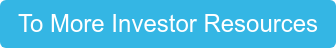If you have ever been on Roofstock’s website, you may have noticed that each property listing has the Current Rent and Cap Rate. But what is cap rate?

## Understanding Cap Rate

Real estate investors use capitalization rate or cap rate to estimate the return on investment for a given property, as well as assess the potential risks of the investment. It is calculated by taking the net operating income (NOI) divided by the market value or purchase price of a property. NOI is a measure of a property's income without taking into consideration taxes or expenses.

To calculate a cap rate, let’s assume a property generates \$14,000 in annual NOI and the market value of the property is \$200,000. Using the cap rate equation, we would take \$14,000 divided by \$200,000, which equals 0.07. Cap rates are expressed as a percentage, so in this example the cap rate would be 7.0% (0.70 * 100 = 7.0%).

## A Closer Look at Cap Rate

As we mentioned, cap rates are used to estimate the return of a given investment and to gauge to the perceived risk of an investment.  For example, let’s say you are looking at two different properties, both generating \$20,000 in annual NOI. If the first property is selling at 5% cap rate, then the seller is asking for \$400,000 (\$20,000 ÷ 5.0% = \$400,000). Compare this to the second property, which is selling at a 10% cap rate. Using the cap rate calculation, the selling price would be \$200,000 (\$20,000 ÷ 10.0% = \$200,000).

Why would two investments with the same NOI differ so drastically in price? Higher cap rates usually are the result of an inferior property location or subpar condition of the property.

## What is a Good Cap Rate?

So, you may be asking what is a good cap rate? And the answer is, it depends on your investment strategy. Keep in mind there are many factors to consider when looking for new investment properties, but for the purposes of this article we are going to focus specifically on cap rate and what it can help you gauge.

When browsing properties on Roofstock, you will see pre-calculated cap rates in the listings ranging from 1.0% to as high as 11.0%. We have already established that properties with higher cap rates represent a potential higher return, but they typically have more “hair” and need more attention. So due diligence is a must in order to determine why the cap rate is higher as this may result in the investor having to invest additional capital to mitigate the issue(s) or is it simply the result of the property’s location.

## Applying Cap Rate to Your Investment Goals

If your investment goal is to spend the lowest amount of money upfront in order to have the highest amount of cash flow, what we call the transitional rental strategy, then definitely consider looking at higher cap rates. In these situations, due diligence is critical in order to determine the root cause of the higher cap rate (poor property condition, location, quality of tenant, etc.) and where additional capital will be required.

On the other hand, if you are investing for the long run and are more interested in your long-term financial gain, what we call the permanent rental strategy, a lower cap rate could help you achieve your goals. These are typically going to be properties in “A” locations with a quality tenant and above average condition (i.e. newly renovated kitchen, new windows, newer roof, etc.).

Essentially, it is important to evaluate your financial situation, goals, and ideal strategy to determine which properties fit your investment thesis. Cap rates are one of several tools that real estate investors use to determine if the investment is worthwhile.